## Looking for your content...Type it here

### GATE Previous Years Papers on Instrumentation Engineering

Q. 1 – Q. 20 Carry One Mark Each.

1) Let A be an real matrix such that = I and y be an dimensional vector.
Then the linear system of equations has

(A) no solution
(B) a unique solution
(C) more than one but finitely many independent solutions
(D) Infinitely many independent solutions

2) Let j = .Then one value of is

(A)
(B) -1
(C)
(D)

3) In full sunlight, a solar cell has a short circuit current of 75 mA and a current of 70 mA for a terminal voltage of 0.6 V with a given load. The Thevenin resistance of the solar cell is.

(A)8Ω
(B) 8.6Ω
(C) 120Ω
(D)240Ω

4) The DC voltage gain in the following circuit is given by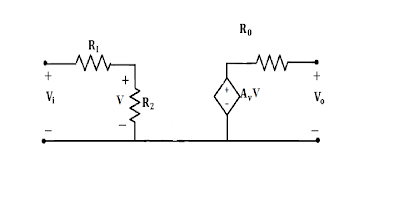(A)
(B)
(C) +
(D)

5) Match the essential amplifier characteristics with the sensing applications given below:

Amplifier Characteristics Sensing Applications

P. Charge amplifier with very low bias current L. Strain gauge in unipolar
and high input impedance

Q. Voltage amplifier with low bias M. Glass electrode pH sensor
current and very high input impedance

R. Voltage amplifier with very high CMRR N. Piezoelectric sensor for
measurement of static force

(A)P – L, Q – M, R – N
(B)P –M, Q – N, R- L
(C)P –N, Q – L, R – M
(D)P – N, Q – M, R – L

6) The figure below shows various configurations of bonding a strain gauge to a cantilever subjected to a bending force FWhich configuration gives the maximum change in resistance for this force?
(A)P
(B)Q
(C) R
(D)All have equal change in resistance

7) When light falls on the photodiode shown in the following circuit, the reverse saturation current of the photodiode changes from 100 μA to 200 μA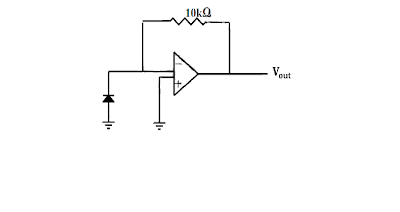Assuming the op – amp to be ideal, the output voltage, of the circuit.
(A)does not change
(B) changes from 1 V to 2V
(C) changes from 2 V to 1 V
(D) changes from -1 V to -2V

8) A 555 astable multivibrator circuit is shown in the figure below: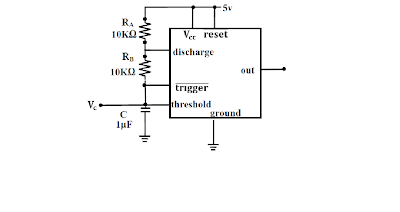If is shorted, the waveform at .9) A Clark oxygen sensor is a

(A)Potentiometric sensor
(B)Amperometric sensor
(C) Conductometric sensor
(D)Magnetostrictive sensor

10) A logic circuit implements the boolean function F = . Y + X. . It is found that the input combination X = Y = 1 can never occur. Taking this into account, a simplified expression for F is given by
(A) + .
(B)X + Z
(C)X + Y
(D)Y + X.

11) A CMOS implementation of a logic gate is shown in the following figure: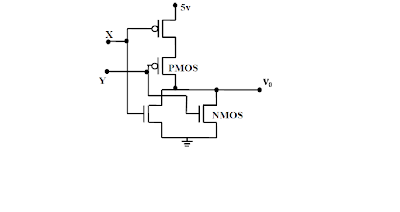The Boolean logic function realized by the circuit is.
(A)AND
(B)NAND
(C)NOR
(D)OR

12) Let x(t) be a continuous-time, real-valued signal band-limited to F Hz. The Nyquist sampling rate, in Hz, for is.
(A)F

(B) 2F
(C) 4F
(D)8F

13) Consider the periodic signal where t is in seconds. Its fundamental frequency, in Hz, is.

(A)20
(B) 40
(C) 100
(D)200

14) A dynamometer type wattmeter with a single scale marked for the smallest power range, has two current ranges, namely, 05 and 010 A as well as two voltage ranges, namely, 0150 V and 0300 V. To carry out a load test on a 230V/115, 1kVA, single phase transformer, the wattmeter is used on the high voltage side. The voltage and current ranges are chosen for maximum utilization of the scale. The multiplying factor to be used in this case is.

(A) 0.5
(B) 1.0
(C) 2.0
(D) 4.0

15) Consider the AC bridge shown in the figure below, with A, L and C having positive finite values.Then.
(A) = 0 if ωL =
(B) = 0 if L = C
(C) = 0 if R =

16) A feedback control system with high gain K, is shown in the figure below:Then the closed loop transfer function is.
(A) sensitive to perturbations in G(s) and H(s)
(B) sensitive to perturbations in G(s) and but not to perturbations in H(s)
(C) sensitive to perturbations in H(s) and but not to perturbations in G(s)
(D) insensitive to perturbations in G(s) and H(s)

17) Consider the following standard state-space description of a linear time-invariant single input single output system: = Ax + Bu, y = Du. Which one of the following statements about the transfer function CANNOT be true if D ≠ 0?.

(A)The system is unstable
(B) The system is strictly proper
(C) The system is low pass
(D)The system is of type zero

18) During intravascular measurement of arterial blood pressure, catheters may be introduced in different configurations, as shown in the figures below:The static pressure will be measured correctly in the configuration (s).
(A)P and Q but not in R
(B) R only
(C)Q only
(D)P and R but not in Q

19) In -washout estimation of lung volume using spirometry, the lung volumes at the beginning and the end of the washout are the same. Let T, V, and F denote temperature, volume and molar fraction (of ) respectively; subscripts S and denote the spirometer and the lung; and and the beginning time and the end time of the experiment, respectively. Then
(A)
(B)
(C)
(D)

20) The dispersion in an X-ray diffractometer, , is given by the expression.
(A)
(B)
(C) 2d sinθ
(D) 2d cosθ

Q. 21 to Q. 75 carry two marks each.

21) The polynomial has.
(A) all real roots
(B) 3 real and 2 complex roots
(C) 1 real and 4 complex roots
(D) all complex roots

22) Let A = 1≤ i, j ≤ n, with n ≥ 3 and = i. j. Then the rank of A is,

(A)0
(B) 1
(C)
(D)n

23) For real x, the maximum value of is

(A)1
(B) e
(C)
(D) ∞

24) Consider the function , where x is real. Then the function f(x) at x = 0 is,

(A) continuous but not differentiable
(B) once differentiable but not twice
(C) twice differentiable but not thrice
(D) thrice differentiable

25) The value of the integral dx dy is.
(A)
(B)
(C) π
(D)

26) For the function of a complex variable z, the point is

(A) a pole of order 3
(B) a pole of order 2
(C) a pole of order 1
(D) not a singularity

27) Assume that the duration in minutes of a telephone conversation follows the exponential distribution . The probability that the conversation will exceed five minutes is

(A)
(B)1 –
(C)
(D)1

28) The boundaryvalue problem yn + λy = 0, y(0) = y(π) = 0 will have non-zero solutions if and only if the values of λ are.
(A) 0, ± 1, ± 2, …..
(B) 1. 2, 3, …..
(C) 1, 4, 9, …..
(D) 1, 9, 25,….

29) Identify the Newton-Raphson iteration scheme for finding the square root of 2.
(A)
(B)
(C)
(D)

30) Consider the linear circuit with and ideal op-amp shown in the figure below.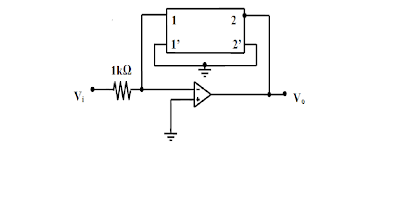The Z-parameters of the two port feedback network are = 11 kΩ and = = 1 kΩ.
(A)+ 110
(B) + 11
(C) - 1
(D) - 120

31) Consider a non-ideal voltage source whose output voltage is measured by a non-ideal voltmeter as shown belowLet be the difference between and the measured voltage The is a function of,
(A) only
(B) only
(C)
(D)

32) Two sensors have measurement errors that are Gaussian distributed with zero means and variances and , respectively. The two sensor measurements and are combined to form the weighted average . Assuming that the measurement errors of the two sensors are uncorrelated, the weighting factor α that yields the smallest error variance of is

(A)
(B)
(C)
(D) 0.5

33) Two square waves of equal period T, but with a time delay τ are applied to a digital circuit whose truth table is shown in the following figure.The high and the low levels of the output of the digital circuit are 5 V and 0 V, respectively. Which one of the following figures shows the correct variation of the average value of the output voltage as a function of τ for 0 ≤ t ≤ (T/2)?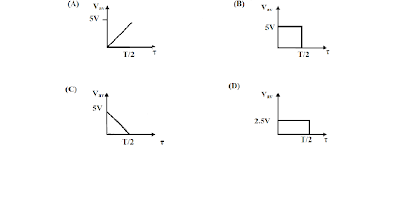In the circuit shown in the following figure, the current through the 1Ω resistor is(A) (1 + 5 cos 2t) A
(B) (5 + cos 2t) A
(C) (1 5 cos 2t) A
(D)6A

35) In the circuit shown in the following figure, the switch is kept closed for a long time and then opened at t = 0.The values of the current i just before opening the switch and just after opening the switch are, respectively
(A) A and 1 A
(B) A and A
(C) 1 A and A
(D)1 A and 1 A

36) Consider the coupled circuit shown belowAt angular frequency ω, this circuit can be represented by the equivalent T – network, shown below.Indicate the correct set of expressions for the impedances of the T – network.

37) A FET source follower is shown in the figure below: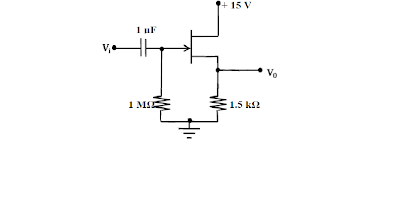The nature of feedback in this circuit is

(A)Positive current
(B)Negative current
(C) Positive voltage
(D)Negative voltage

38) In the circuit shown below,The β of the transistor and are, respectively
(A) 19 and 2.8 V
(B) 19 and 4.7 V
(C) 38 and 2.8 V
(D) 38 and 4.7 V

39) A well of cross – sectional area is connected to an inclined tube of cross – sectional area to form a differential pressure gauge as shown in the figure below. When the common liquid level is denoted by A. When , the liquid level in the well is depressed to B, and the level in the tube rises by along its length such that the difference in the tube and well levels is .The angle of inclination θ of the tube with the horizontal is
(A)
(B)
(C)
(D)

40) The accompanying figure shows a VERTICAL venturimeter with upward water flow. When the measured static pressure difference, , between the inlet and the throat is 30 kPa, the flow rate is found to be 50 litres per second. Assume that the coefficient of discharge remains the same. When the flow rate, in litres per second, is(A) 33.3
(B) 39.3
(C) 40.8
(D) 54.2

41) A thermometer with time constant τ, initially at the ambient temperature, is used to measure the temperature of a liquid in a bath. The excess temperature of the thermometer and the liquid over the ambient are and (t), respectively, where t denotes the time. If (t), = kt, where k is a constant, the steady state error, defined as (t)], is.

(A) ∞
(B) 0
(C) –
(D)

42) In a laminar flow experiment, Fluid A is pumped through a straight tube and the volumetric flow rate and pressure drop per unit length are recorded. In a second straight tube having twice the internal diameter of the first one, Fluid B recorded the same pressure drop per unit length at the same volumetric flow rate. Assuming fully developed flow conditions in the tubes, the ratio of the dynamic viscosity of Fluid B to that of Fluid A is,

(A)16
(B) 32
(C) 64
(D)128

43) A thermistor has a resistance of 10 kΩ at C and 1 kΩ at C. The range of operation is CC. The excitation voltage is 5V and a series resistor of 1 kΩ is connected to the thermistor.The power dissipated in the thermistor at C is
(A) 4.0 mW
(B) 4.7 mW
(C) 5.4 mW
(D) 6.1 mW

44) A measurement system for the rotational speed of a motor is shown in the figure below. The system consists of an opaque disk attached to the motor shaft with a hole as shown. A light source and a photodetector are placed on two sides of the disk so that whenever the hole cross the light path, the photodetector receives light through the hole. The photodetector circuit is also shown below. Assume sufficient light intensity and TTL logic levels for the inverter.The output the photodetector is a
(A)Triangular wave
(B) Square wave with 50% duty cycle
(C) Rectangular wave with duty cycle close to unity
(D)Rectangular wave with duty cycle to zero

45) A pH electrode, being used at C, has a source resistance of Ω. The electrode obeys the Nernst equation perfectly. The electronic voltmeter, with which the potential is being measured, has an input impedance of Ω and a gain of 100. If the pH of the analyte changes from 6.5 to 7.8, the change in voltage observed on the voltmeter is
(A) less than 6.8 V
(B) between 6.8 V and 7.19 V
(C) between 7.2 V and 7.49 V
(D)greater than 7.49 V

46) The figure shows a single op-amp differential amplifier circuit.Which one of the following statements about the output is correct?
(A)
(B) 95 mV < ≤ 98 mV (C) 98 mV < ≤ 101 mV (D) > 101 mV

47) The three transistors in the circuit shown below are identical, with = 0.7 V and β = 100.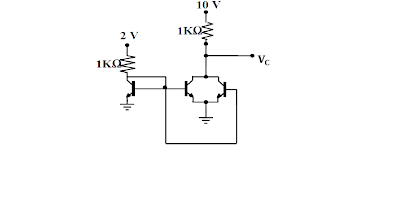The voltage is
(A) 0.2 V
(B) 2 V
(C) 7.4 V
(D)10 V

48) The input signal shown in the figure below is fed to a Schmitt trigger. The signal has a square wave amplitude of 6 V pp. It is corrupted by an additive high frequency noise of amplitude 8 V.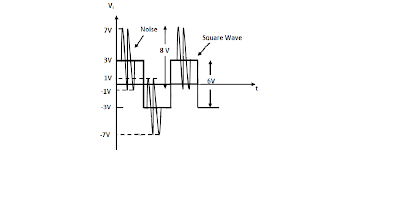Which one of the following is an appropriate choice for the upper and lower trip points of the Schmitt trigger to recover a square wave of the same frequency from the corrupted input signal

(A)± 8.0 V
(B) ± 2.0 V
(C) ± 0.5 V
(D)0V

49) Consider the circuit shown belowThe correct frequency response of the circuit is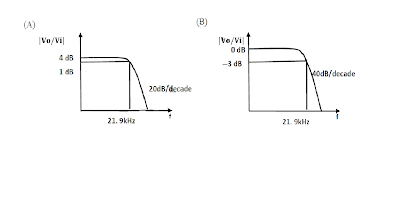50) Let X and Y = be unsigned 2bit numbers. The function F = 1 if X > Y and F = 0 otherwise. The minimized sum of products expression for F is
(A) + ..
(B) + +
(C) + +
(D)

51) A MUX circuit shown in the figure below implements a logic function .
The correct expression for is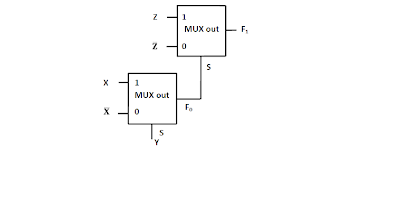(A)
(B)
(C)
(D)

52) A sequential circuit is shown in the figure below. Let the state of the circuit be encoded as. The notation X→Y implies that state Y is reachable from state X in a finite number of clock transitions.Identify the INCORRECT statement.
(A)01 → 00
(B) 11 → 01
(C) 01 → 11
(D)01 → 10

53) A snapshot of the address, date and control buses of an 8085 microprocessor executing a program is given below:

 Address 2020H Data 24H Logic high Logic high Logic Low
The assembly language instruction being executed is
(A) IN 24H
(B) IN 20H
(C)OUT 24H
(D)OUT 20H

54) The circuit shown in the figure below works as a 2bit analog to digital converter for 0 ≤ ≤ 3 V.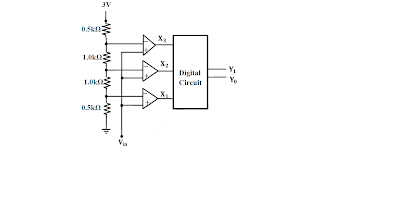The MSB of the output , expressed as a Boolean function of the inputs ,, , is given by
(A)
(B)
(C)
(D) +

55) 8bit signed integers in 2’s complement form are read into the accumulator of an 8085 microprocessor from an I/O port using the following assembly language program segment with symbolic addresses

BEGIN: IN PORT
RAL
JNC BEGIN
RAR
END: HLT

This program
(A)Halts upon reading a negative number
(B)Halts upon reading a positive number
(D)Never halts

56) In the circuit shown in the figure, the input signal is = 5+3 cos ωt.The steady state output is expressed as , the values of P and Q are
(A)P = 0 and Q = 6 /
(B) P = 0 and Q = 3 /
(C) P = 5 and Q = 6 /
(D)P = 5 and Q = 3

57) The signals x(t) and h(t) shown in the figures are convolved to yield y(t)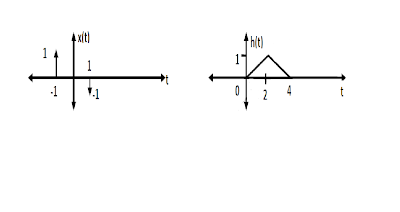Which one of the following figures represents the output y(t)?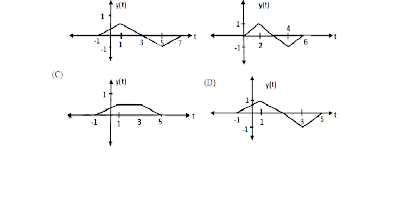58) Consider the discretetime signal u(n), where u(n) =. Define the signal equals

(A)
(B)
(C)
(D)3

59) Let the signal x(t) have the Fourier transform X(ω). Consider the signal where is an arbitrary delay. The magnitude of the Fourier transform of y(t) is given by the expression
(A)
(B) ω
(C)
(D)

60) In the circuit shown below the switch (S) is closed whenever the input voltage is positive and open otherwise.The circuit is a
(A)Low pass filter
(B) Level shifter
(C)Modulator
(D)Precision rectifier

61) The linear sweep for the time base in an oscilloscope has deviation from its nominal waveform. The nominal (dashed line) and actual (solid line) sweep waveforms are shown in the following figure.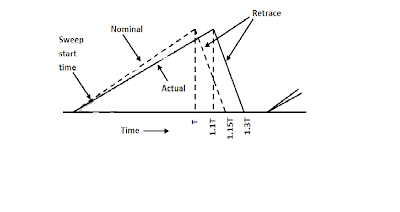A 5V pp sine wave with a frequency of 1 kHz will be measured on the oscilloscope as a sine wave with
(A) 4.45 V pp and 1 kHz frequency
(B) 5 V pp and 1kHz frequency
(C) 5 V pp and 1.1 kHz frequency
(D)5 V pp and 1.15 kHz frequency

62) The pulse width T of an asynchronous pulse is measured by a counter with an edge-triggered clock of known frequency as shown in the figure below:The pulse, whose width is to be measured, is applied to the Enable pin of the counter. The counter counts while the Enable is high and is held reset to zero otherwise. The counter output is latched by the negative edge of the Enable signal. The measured pulse width is taken to be N times the clock period, where N is the count reached at the end of a count cycle.Assuming no overflow, the measurement error will be limited to x% of T if
(A)T
(B)T
(D)T

63) The figure shows a potentiometer of total resistance with a sliding contact.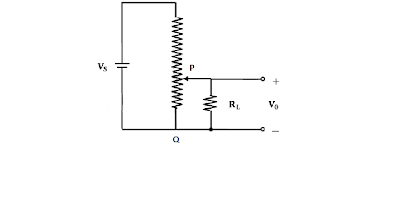The resistance between the points P and Q of the potentiometer at the position of the contact shown is and the voltage ratio at this point is 0.5. If the ratio =1, the ratio is
(A)
(B)
(C) 1+
(D)1+

64) Consider the triangular wave generator shown below.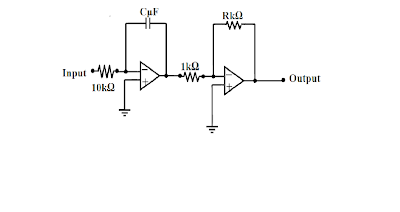65)Consider the triangular wave generator shown below.
Assume that the opamps are ideal and have ± 12 V power supply. If the input is a ± 5 V 50 Hz square wave of duty cycle 50%, the condition that results in a triangular wave of peak to peak amplitude 5 V and frequency 50 Hz at the output is
(A)RC = 1
(B)
(C)
(D)

65) Two signals of peak – to – peak voltages 5 V and 2V are being fed to Channel 1 and Channel 2, respectively, of an oscilloscope with a single time base. The vertical sensitivity of both channels is 1 V/ division. The two sine waves have identical frequency and phase. The trigger is on manual mode the triggers at a level of +1.25 V on Channel 1, as shown in the figure below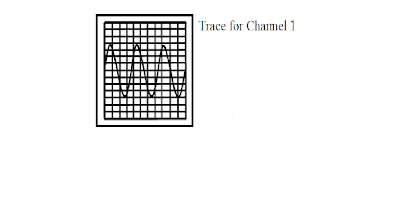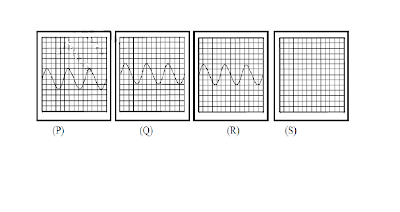Which of the following figures correctly depicts the trace seen in channel 2

(A)P
(B)Q
(C) R
(D)S

66) A chamber is heated with a heater of maximum power rating of 1000 W. The process transfer relationship between the steady-state heater power and chamber temperature is assumed to be linear with a slope of 2 Watts c. The heater power is the sum of the output of a proportional controller having a proportional band of 200% and a constant offset of 500W. The temperature achieved for a set point of C is
(A) C
(B) C
(C) C
(D) C

67) A Cascade control system with proportional controllers is shown below.Theoretically, the largest values of the gains and that can be set without causing instability of the closed loop system are
(A) 10 and 100
(B) 100 and 10
(C) 10 and 10
(D) α and

68) The ECG of a patient is being recorded using the three standard frontal plane leads. If the cardiac vector is oriented at an angle of 45 degrees to Lead I and has a magnitude of 3mV, the voltages seen on Leads I, II and III are

(A) 1.50, 2.27 and 0.77 mV
(B) 2.12, 0.77 and 2.89 mV
(C) 2.12, 3.15 and 1.03 mV
(D) 2.12, 2.89 and 0.77 mV

69) Light of intensity is equally divided and passed through 2 cuvettes and containing an analyte at concentrations c and 0.5c, respectively. The corresponding path lengths in and are 4cm and 1cm. The cross-sectional areas are 1 and 3 , respectively. The ratio of the absorbances in and is
(A) 1.5
(B) 8/3
(C) 3
(D)8

70) The H-D curves of two X-ray films, and , are shown below.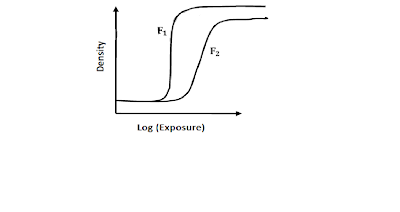The curves show that, for the same change in exposure, has

(A) higher contrast than but the same fog level
(B) lower contrast than but the same fog level
(C) higher contrast and higher fog level than
(D) lower contrast than but higher fog level

Common Data Questions

Common Data for Questions 71, 72, 73 :

Consider the op-amp circuit shown in the figure below.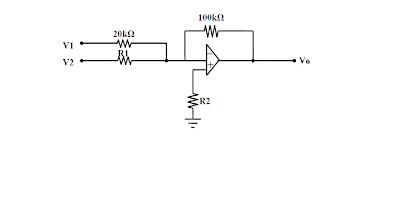71) 71. If V1= 0.2 V, V2 = 0.6 V and V0 = 7V, and the op-amp is ideal, the value of is

(A)5kΩ
(B) 10kΩ
(C) 15kΩ
(D)20kΩ

72) Let = = sin 2πf t and = 20kΩ. The op-amp has a slew rate of 0.5 V/μs with its other parameters being ideal. The values of and f for which the amplifier output will have no distortion are, respectively

(A) 0.1 V and 300 kHz
(B) 0.5 V and 300 kHz
(C) 0.1 V and 30 kHz

73) Let = = 0 and = 20kΩ. Assume that the op-amp is ideal except for a non-zero input bias current. What is the value of for the output voltage of the op-amp to be zero?
(A) 2.2kΩ
(B) 9.1kΩ
(C) 20kΩ
(D)100kΩ

Common Data for Questions 74,75:
The following figure represents a proportional control scheme of a first order system with transportation lag.74) The angular frequency in radians/s at which the loop phase lag becomes is
(A) 0.408
(B) 0.818
(C) 1.56
(D) 2.03

75) The steady state error for a unit step input when the gain = 1 is
(A)
(B)
(C) 1
(D)2

Linked Answer Questions : Q. 76 to Q. 85 carry two marks each.

Blood flow through a straight segment of an artery is measured using a Doppler flowmeter. The probe is oriented at an angle of 45 degrees to the longitudinal axis of the artery. It is known that Doppler shift is proportional to the velocity of the blood in the direction of propagation of sound from the probe and the frequency generated from the probe. The velocity of sound in blood is 1500 m/s and the maximum blood flow velocity to be measured is 110 cm/s.

76) Signal processing limitations constrain the maximum Doppler shift to be less than 3 kHz. The maximum source frequency (probe output), to the nearest MHz, should be

(A)2 MHz
(B) 3 MHz
(C) 5 MHz
(D)10 MHz

77) In Q.76, if the piezoelectric crystal of the probe is made of quartz (Young’s modulus, Y = 80 × , ρ = 2.65 × kg.), the maximum thickness of the crystal required to resonate at the frequency determined above, to the nearest mm, is
(A)1 mm
(B) 2 mm
(C) 3 mm
(D)4 mm

Consider the circuit shown in the following figure.78) The correct input-output relationship between Y and (,) is

79) The D flip-flops are initialized to = 000. After 1 clock cycle, is equal to
(A)011
(B) 010
(C) 100
(D)101

The numerical aperture of a step index fiber in air (refractive index = 1), is 0.39. The diameter of the core is 200 μm.

80) The angle of acceptance when the fiber is used in water (refractive index = 1.33) is closest to
(A)
(B)
(C)
(D)

81) Two experiments are conducted in which light is launched into the fiber from a uniformly distributed planar source kept 5 mm away from the tip. In the first experiment () both the source and the fiber are in air. In the second experiment () both the source and the fiber are in water. Neglecting absorption in the medium between the source and the tip, the ratio of the amount of light coupled into the fiber in to the amount of light coupled into the fiber in is closest to
(A) 0.5
(B) 1.0
(C) 1.4
(D) 1.9

A push-pull capacitive displacement transducer is interfaced to a differential amplifier and an ADC as shown in the figure below.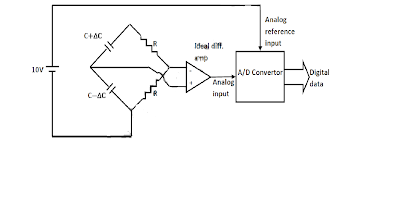Note that the bridge supply and the analog reference input for the ADC are derived from the same 10 V DC source.

82) The change in capacitance for full scale displacement is ±5% for the capacitors. The gain of the differential amplifier for utilization of the full range of the ADC (which is ±10V) is
(A)10
(B) 20
(C) 30
(D)40

83) the supply voltage to the bridge decreases by 5%, the sensitivity of the measurement system
(A) decreases by 5%
(B) does not change
(C) increases by 5%
(D) increases by 200%

A transfer function with unity DC gain has three poles at 1, 2, and 3 and no finite zeros. A plant with this transfer function is connected with a proportional controller of gain K in the forward path, in a unity feedback configuration.

84. The transfer function is

(A)
(B)
(C)
(D)

85) If the root locus plot of the closed loop system passes through the points ± j, the maximum value of K for stability of the unity feedback closed loop system is

(A)
(B) 6
(C) 10
(D)6